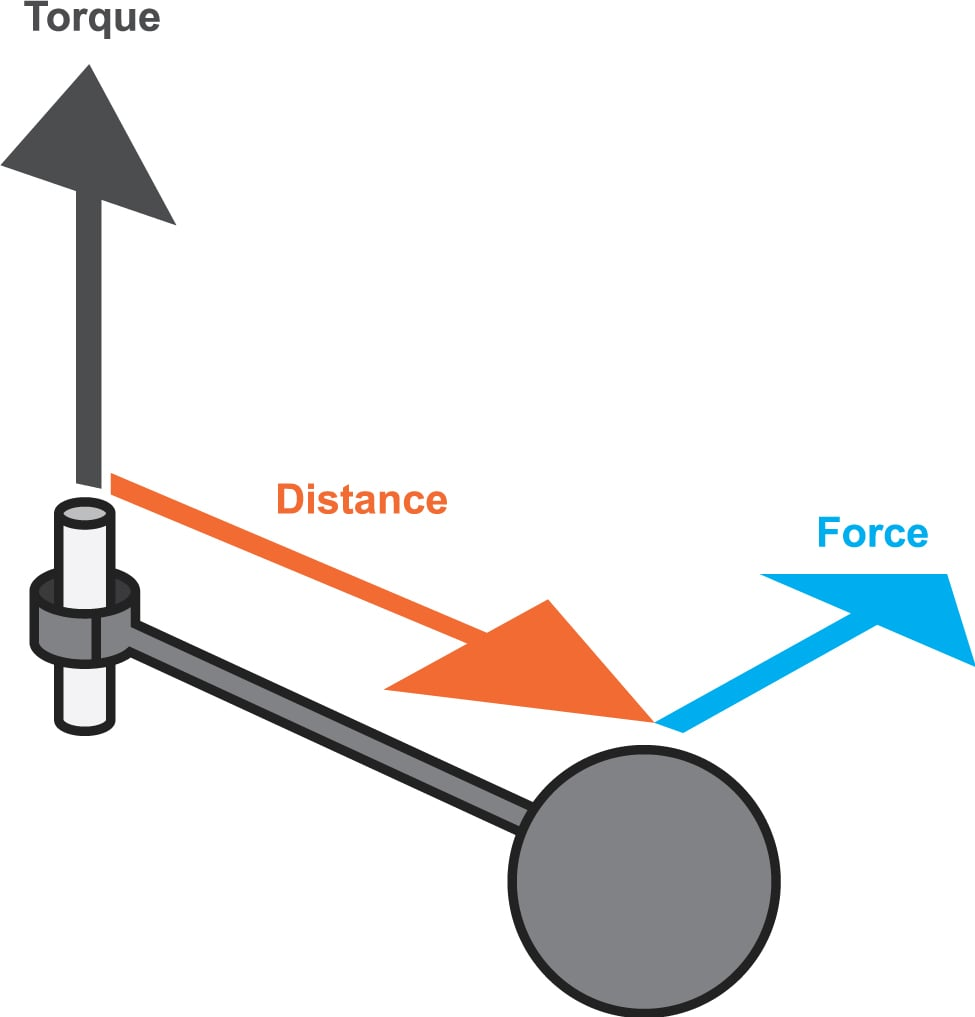# Torque Conversion Calculator

## A tool designed to convert between units of torque

### OverviewTorque is the measure of the tendency of a force to rotate an object about an axis. The SI unit for torque is Newton-meters. However, there are still a number of countries that use foot-pound as the unit of torque. This calculator is designed to convert between torque units.

### Equation

$$\tau_{Nm} = 1.355818\tau_{ft-lb}$$

Where:

τNm = torque in Newton-meters

τft−lb = torque in foot-pounds

### Applications

The concept of torque is significant in a lot of areas in electrical engineering. One particular instance is when an electric dipole experiences a rotational force from an electric field. The torque experienced by that electric dipole is the cross product between the dipole moment and the electric field.

The torque of a motor is an important parameter often considered by engineers. By comparing the torque (output), with the voltage (input), the efficiency of a motor can be calculated. An efficient motor means the device does not consume or waste a lot of power when in operation.• A
albertopyhy9 October 13, 2021

I would like a version that I can have on my computer because I don’t always have a connection.

Cheers!
tileinstallationtampafl.com

Like.
•steftje November 09, 2021

the picture shows this metric formula,  19kg*58.5cm/100=11.115 kgf*9,807=109nm torque
nm / 9,807*100/ length = kg   standard mathematics not sience mathematics, read from left to right.  so the kg is how hard you push or pull on the wrench and the nm is the center.  same for a motor if its giving torque and the sprocket beam size, how many kg it can pul.

Like.
• M
Marie143 March 01, 2022

Thanks for the wonderful share. Your article has proved your hard work and experience you have got in this field. Brilliant .I love it reading.